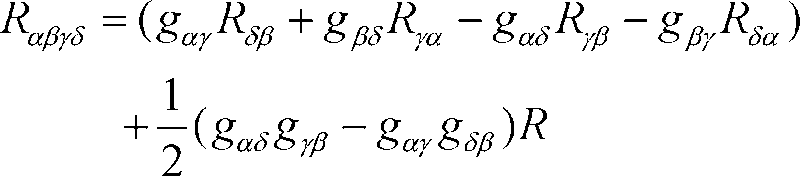# Weyl tensor on 3-dimensional manifold

sroeyz
Hello, I wish to show that on 3-dimensional manifolds, the weyl tensor vanishes.
In other words, I want to show that the curvature tensor, the ricci tensor and curvature scalar hold the relationPlease, if anyone knows how I can prove this relation or refer to a place which proves the relation, I will be most grateful.

Staff Emeritus
Homework Helper
Gold Member
One should be able to count the number of algebraically independent components of the Riemann tensor in n-dimensions... then compare to the number for Ricci.

sroeyz
I know the final answer is that the number of independent components is
(n^2(n^2-1))/12

Can you please show me how to reach this result?

Staff Emeritus
I'll take a shot at doing the Riemann.

Consider its symmetries: it's anti-symmetric in the first two indicies, so

R_abcd = -R_bacd

thus if a=b, we know that the Riemann is zero

Similarly, IF the Riemann is derived from a metric

R_abcd = -R_abdc, i.e. it's anti-symmetric in the last two indices.

I think we need to assume that the Riemann is a Riemann derived from a metric...

So far we have basically shown by symmetry that the Riemann must be

R(u)(v), where u and v are anti-symmetric rank 2 tensors, aka two forms.

How many 2-forms do we have in 3-d space? We have

x^y, x^z, and y^z - a total of three. Let's call them p, q, and s

The order of the two-forms doesn't matter because

R_abcd = R_cdab (symmetry under exchange of front pair with back pair)

So our possibilities are so far

pp, pq, ps, qq, qs, ss

That's 6, which is the right answer. I can see that there aren't any completely anti-symmetric terms to eliminate in only three dimensions, therfore

R_[abcd]=0

doesn't add any constraints. An open question:

R_a[bcd]=0

is the last remaining symmetry, it must also not contribute??

sroeyz said:
Hello, I wish to show that on 3-dimensional manifolds, the weyl tensor vanishes.
In other words, I want to show that the curvature tensor, the ricci tensor and curvature scalar hold the relation

View attachment 7457

Please, if anyone knows how I can prove this relation or refer to a place which proves the relation, I will be most grateful.

Weyl tensor possesses the same symmetries of the Reimann tensor, and satisfies the n(n+1)/2 equations;

$$C_{abc}{}^{b} = 0$$

Almost all books on tensor calculus prove that the number of independent components of Reimann tensor is equal to;

$$\frac{n^{2}(n^{2} - 1)}{12}$$

So, in n-dimensional manifold, the number of independent components
of the Weyl tensor is equal to;

$$\frac{n^{2}(n^{2} - 1)}{12} - \frac{n(n+1)}{2}$$

Thus, for n=3, Weyl tensor has zero number of components, i.e

$$C_{abcd} = 0$$

regards

sam

Last edited:
•Spinnor
sroeyz said:
View attachment 7457

Please, if anyone knows how I can prove this relation or refer to a place which proves the relation, I will be most grateful.

$$R_{ac}=3R_{ac}-R_{ac}-R_{ac}+Rg_{ac}- \frac{R}{2}(3g_{ac}-g_{ac})$$

then, rewrite it in 3D, i.e put;

$$3=g_{a}^{a}= \delta_{a}^{a}$$

So,

$$g^{bd}R_{abcd}=R_{ac}g_{b}^{b}-R_{bc}g_{a}^{b}-R_{ad}g_{c}^{d}+R_{bd}g^{bd}g_{ac}-(1/2)R(g_{ac}g_{b}^{b}-g_{bc}g_{a}^{b})$$

or;

$$g^{bd}R_{abcd}=g^{bd}[R_{ac}g_{bd}-R_{ad}g_{bc}+R_{bd}g_{ac}-R_{bc}g_{ad}+(1/2)R(g_{ac}g_{bd}-g_{ad}g_{bc})]$$

Now, in 3D, Reimann and Ricci have the same number of independent components (6 each). Therefore the Reimann tensor is determined completely by the Ricci tensor and the above equation gives;

$$R_{abcd}=R_{ac}g_{bd}-R_{ad}g_{bc}+R_{bd}g_{ac}-R_{bc}g_{ad}-(1/2)R(g_{ac}g_{bd}-g_{ad}g_{bc})$$

regards

sam

•Spinnor